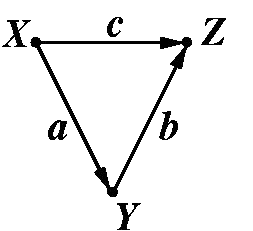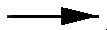# Causal Analysis in Theory and Practice

## October 21, 2001

### Indirect Effects

Filed under: Indirect effects — moderator @ 12:00 am

From Melanie Wall, University of Minnesota:

I am teaching a course in latent variable modeling (to biostatistics and other public health students) and was yesterday introducing path analysis concepts including direct and indirect effects.

I showed them how to calculate indirect effects by taking the product of direct paths. Then a student asked about how to interpret the indirect effect and I gave the answer that I always give, that the indirect effect ab (in the following simple model) is the effect that a change in x has on Z through its relationship with Y.After chewing on this for a second, the student asked the following:

Student: "The interpretation of the b path is: b is the increase we would see in Z given a unit increase in Y while holding X fixed, right?"

Me: "That's right"

Student: "Then what is being held constant when we interpret an indirect effect?"

Me: "Not sure what you mean"

Student: "You said the interpretation of the indirect effect ab is: ab is the increase we would see in Z given a one unit increase in X through its causal effect on Y. But since b (the direct effect from Y to Z) requires X to be held constant how can it be used in a calculation that is also requiring X to change one unit"

Me: "Hmm. Very good question, I'm not sure I have a good answer for you. In the case where the direct path from XZ is zero I think we have no problem since the relationship between Y and Z then has nothing to do with X. But you are right, here if "c" is non-zero then we must interpret b as the effect of Y on Z when X is held constant. I understand that this sounds like it conflicts with the interpretation of the ab indirect effect where we are examining what a change in X will cause. How about I get back to you. As I have told you before, the calculations here aren't hard, its trying to truly understand what your model means that's hard."

## 1 Comment »

1. Dear Melanie,
Congratulate your student on his/her inquisitive mind. The answer can be formulated rather simply:

The indirect effect of X on Z is the increase we would see in Z while holding X constant and increasing Y to whatever value Y would attain under a unit increase of X.

Policy questions that depend on indirect effects are discussed in my paper R-273 (Direct and Indirect Effects).

This question represents one of several areas where standard SEM education should be reformed. Invariably, SEM textbook give but a cursory mention (if any) of the interpretation of structural parameters as effect coefficients and, once mentioned, this interpretation is not taken very seriously by authors and teachers. The bulk of SEM education focuses on techniques of statistical estimation and model fitting, and one can hardly find a serious discussion of what the model means, once it is fitted and estimated.

The weakness of this educational tradition surfaces when inquisitive students ask questions that deviate slightly from standard LISREL routines, the answers to which depend critically on the interpretation of structural coefficients and structural equations.

For example:

1. Why should we define the total effect the way we do? (i.e., as sum of products of certain direct effects.)&nbsp; Is this an arbitrary definition, or is it compelled by the experimental setups relative to which total and direct effects are defined?
2. Why should we define the indirect effect as the difference between the total and direct effect?
3. How can we define direct effect in nonlinear systems or in systems involving dichotomous variables?
4. How should we, in a meaningful way, define effects in systems involving feedback loops (i.e. reciprocal causation) so as to avoid the pitfalls of erroneous definitions quoted in SEM textbooks?&nbsp; (see Causality, p. 164).
5. Would our assessment of direct and total effects remain the same if we were to take some measurements prior to implementing the action whose effect we attempt to estimate?

Readers of Causality will be pleased to note that many of these questions can now be given formal answers.

Best wishes,
========Judea Pearl

Comment by judea — October 24, 2001 @ 12:00 am